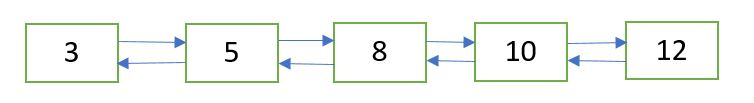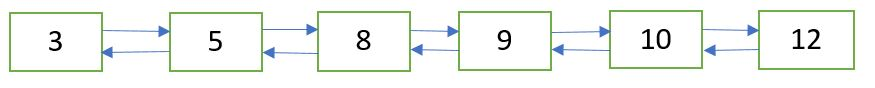Related Articles
Insert value in sorted way in a sorted doubly linked list
• Difficulty Level : Easy
• Last Updated : 31 Aug, 2020

Given a sorted doubly linked list and a value to insert, write a function to insert the value in sorted way.Doubly Linked List after insertion of 9Algorithm:
Let input doubly linked list is sorted in increasing order.
New node passed to the function contains data in the data part and previous and next link are set to NULL.

```sortedInsert(head_ref, newNode)

newNode->next->prev = newNode

else
while (current->next != NULL and
current->next->data < newNode->data)
current = current->next

newNode->next = current->next
if current->next != NULL
newNode->next->prev = newNode

current->next = newNode
newNode->prev = current
```

## C++

 `// C++ implementation to insert value in sorted way ` `// in a sorted doubly linked list ` `#include ` ` `  `using` `namespace` `std; ` ` `  `// Node of a doubly linked list ` `struct` `Node { ` `    ``int` `data; ` `    ``struct` `Node* prev, *next; ` `}; ` ` `  `// function to create and return a new node ` `// of a doubly linked list ` `struct` `Node* getNode(``int` `data) ` `{ ` `    ``// allocate node ` `    ``struct` `Node* newNode =  ` `        ``(``struct` `Node*)``malloc``(``sizeof``(``struct` `Node)); ` ` `  `    ``// put in the data ` `    ``newNode->data = data; ` `    ``newNode->prev = newNode->next = NULL; ` `    ``return` `newNode; ` `} ` ` `  `// function to insert a new node in sorted way in ` `// a sorted doubly linked list ` `void` `sortedInsert(``struct` `Node** head_ref, ``struct` `Node* newNode) ` `{ ` `    ``struct` `Node* current; ` ` `  `    ``// if list is empty ` `    ``if` `(*head_ref == NULL) ` `        ``*head_ref = newNode; ` ` `  `    ``// if the node is to be inserted at the beginning ` `    ``// of the doubly linked list ` `    ``else` `if` `((*head_ref)->data >= newNode->data) { ` `        ``newNode->next = *head_ref; ` `        ``newNode->next->prev = newNode; ` `        ``*head_ref = newNode; ` `    ``} ` ` `  `    ``else` `{ ` `        ``current = *head_ref; ` ` `  `        ``// locate the node after which the new node ` `        ``// is to be inserted ` `        ``while` `(current->next != NULL &&  ` `               ``current->next->data < newNode->data) ` `            ``current = current->next; ` ` `  `        ``/* Make the appropriate links */` `        ``newNode->next = current->next; ` ` `  `        ``// if the new node is not inserted ` `        ``// at the end of the list ` `        ``if` `(current->next != NULL) ` `            ``newNode->next->prev = newNode; ` ` `  `        ``current->next = newNode; ` `        ``newNode->prev = current; ` `    ``} ` `} ` ` `  `// function to print the doubly linked list ` `void` `printList(``struct` `Node* head) ` `{ ` `    ``while` `(head != NULL) { ` `        ``cout << head->data << ``" "``; ` `        ``head = head->next; ` `    ``} ` `} ` ` `  `// Driver program to test above ` `int` `main() ` `{ ` `    ``/* start with the empty doubly linked list */` `    ``struct` `Node* head = NULL; ` ` `  `    ``// insert the following nodes in sorted way ` `    ``struct` `Node* new_node = getNode(8); ` `    ``sortedInsert(&head, new_node); ` `    ``new_node = getNode(5); ` `    ``sortedInsert(&head, new_node); ` `    ``new_node = getNode(3); ` `    ``sortedInsert(&head, new_node); ` `    ``new_node = getNode(10); ` `    ``sortedInsert(&head, new_node); ` `    ``new_node = getNode(12); ` `    ``sortedInsert(&head, new_node); ` `    ``new_node = getNode(9); ` `    ``sortedInsert(&head, new_node); ` ` `  `    ``cout << ``"Created Doubly Linked Listn"``; ` `    ``printList(head); ` `    ``return` `0; ` `} `

## Java

 `// Java implementation to insert value in sorted way  ` `// in a sorted doubly linked list  ` `import` `java.io.*; ` `import` `java.util.*; ` ` `  `// Node of a doubly linked list ` `class` `Node  ` `{ ` `    ``int` `data; ` `    ``Node next, prev; ` `} ` ` `  `class` `GFG ` `{ ` ` `  `    ``// function to create and return a new node  ` `    ``// of a doubly linked list  ` `    ``static` `Node getNode(``int` `data)  ` `    ``{ ` `            ``// allocate node  ` `            ``Node newNode = ``new` `Node(); ` ` `  `            ``// put in the data  ` `            ``newNode.data = data;  ` `            ``newNode.prev = newNode.next = ``null``;  ` `            ``return` `newNode;  ` ` `  `    ``} ` ` `  `    ``// function to insert a new node in sorted way in  ` `    ``// a sorted doubly linked list  ` `    ``static` `Node sortedInsert(Node head, Node newNode) ` `    ``{ ` `            ``Node current; ` ` `  `            ``// if list is empty  ` `            ``if` `(head == ``null``) ` `                ``head = newNode;  ` ` `  `            ``// if the node is to be inserted at the beginning  ` `            ``// of the doubly linked list  ` `            ``else` `if` `(head.data >= newNode.data) ` `            ``{ ` `                ``newNode.next = head; ` `                ``newNode.next.prev = newNode; ` `                ``head = newNode; ` `            ``} ` ` `  `            ``else`  `            ``{ ` `                ``current = head; ` ` `  `                ``// locate the node after which the new node  ` `                ``// is to be inserted  ` `                ``while` `(current.next != ``null` `&&  ` `                        ``current.next.data < newNode.data)  ` `                    ``current = current.next; ` ` `  `                ``/* Make the appropriate links */` `                ``newNode.next = current.next; ` ` `  `                ``// if the new node is not inserted  ` `                ``// at the end of the list ` `                ``if` `(current.next != ``null``)  ` `                    ``newNode.next.prev = newNode;  ` ` `  `                ``current.next = newNode;  ` `                ``newNode.prev = current;  ` `             `  `            ``} ` `            ``return` `head; ` `    ``} ` ` `  `    ``// function to print the doubly linked list  ` `    ``static` `void` `printList(Node head) ` `    ``{ ` `            ``while` `(head != ``null``)  ` `            ``{ ` `                    ``System.out.print(head.data + ``" "``); ` `                    ``head = head.next; ` `            ``} ` ` `  `    ``} ` ` `  `    ``// Driver code ` `    ``public` `static` `void` `main(String args[]) ` `    ``{ ` `            ``/* start with the empty doubly linked list */` `            ``Node head = ``null``; ` ` `  `            ``// insert the following nodes in sorted way ` `            ``Node new_node = getNode(``8``); ` `            ``head = sortedInsert(head, new_node);  ` `            ``new_node = getNode(``5``);  ` `            ``head = sortedInsert(head, new_node);  ` `            ``new_node = getNode(``3``);  ` `            ``head = sortedInsert(head, new_node);  ` `            ``new_node = getNode(``10``);  ` `            ``head = sortedInsert(head, new_node);  ` `            ``new_node = getNode(``12``);  ` `            ``head = sortedInsert(head, new_node);  ` `            ``new_node = getNode(``9``);  ` `            ``head = sortedInsert(head, new_node); ` ` `  `            ``System.out.println(``"Created Doubly Linked List"``); ` `            ``printList(head); ` `    ``} ` `} ` ` `  `// This code is contributed by rachana soma `

## Python3

 `# Python3 implementation to insert ` `# value in sorted way in a sorted ` `# doubly linked list ` ` `  `# Node of a doubly linked list ` `class` `Node: ` ` `  `    ``# Constructor to initialize  ` `    ``# the node object ` `    ``def` `__init__(``self``, data): ` `         `  `        ``self``.data ``=` `data ` `        ``self``.``next` `=` `None` `        ``self``.prev ``=` `None` ` `  `class` `DoublyLinkedList: ` ` `  `    ``# Function to initialize head ` `    ``def` `__init__(``self``): ` `         `  `        ``self``.head ``=` `None` ` `  `    ``# Function to create and return a  ` `    ``# new node of a doubly linked list ` `    ``def` `getNode(``self``, data): ` `         `  `        ``return` `Node(data) ` ` `  `    ``# Function to insert a new node in  ` `    ``# sorted way in a sorted doubly linked list ` `    ``def` `sortedInsert(``self``, data): ` `         `  `        ``new_node ``=` `self``.getNode(data) ` ` `  `        ``# If the list is empty ` `        ``if` `self``.head ``is` `None``: ` `            ``self``.head ``=` `new_node ` `         `  `        ``# If the node is to be inserted at   ` `        ``# the beginning of the doubly linked list  ` `        ``elif` `self``.head.data >``=` `new_node.data: ` `            ``new_node.``next` `=` `self``.head ` `            ``new_node.``next``.prev ``=` `new_node ` `            ``self``.head ``=` `new_node ` `        ``else``:  ` `            ``current ``=` `self``.head ` ` `  `            ``# Locate the node after which  ` `            ``# the new node  is to be inserted ` `            ``while` `((current.``next` `is` `not` `None``) ``and`  `                   ``(current.``next``.data < new_node.data)): ` `                ``current ``=` `current.``next` `     `  `            ``# Make the appropriate links ` `            ``new_node.``next` `=` `current.``next` ` `  `            ``# If the new node is not inserted  ` `            ``# at the end of the list ` `            ``if` `current.``next` `is` `not` `None``: ` `                ``new_node.``next``.prev ``=` `new_node ` ` `  `            ``current.``next` `=` `new_node ` `            ``new_node.prev ``=` `current ` `     `  `    ``# Function to print the doubly linked list ` `    ``def` `printList(``self``): ` ` `  `        ``node ``=` `self``.head ` `        ``while` `node: ` `            ``print``(``str``(node.data), end ``=` `" "``) ` `            ``node ``=` `node.``next` ` `  `# Driver code ` `if` `__name__ ``=``=` `'__main__'``: ` ` `  `    ``# Insert the following nodes ` `    ``# in sorted way  ` `    ``llist ``=` `DoublyLinkedList() ` `    ``llist.sortedInsert(``8``) ` `    ``llist.sortedInsert(``5``) ` `    ``llist.sortedInsert(``3``) ` `    ``llist.sortedInsert(``10``) ` `    ``llist.sortedInsert(``12``) ` `    ``llist.sortedInsert(``9``) ` `     `  `    ``print``(``"Created Doubly Linked List"``) ` `    ``llist.printList() ` ` `  `# This code is contributed by Siddhartha Pramanik `

## C#

 `// C# implementation to insert value in sorted way  ` `// in a sorted doubly linked list  ` `using` `System;  ` ` `  `// Node of a doubly linked list  ` `public` `class` `Node  ` `{  ` `    ``public` `int` `data;  ` `    ``public` `Node next, prev;  ` `}  ` ` `  `class` `GFG  ` `{  ` ` `  `    ``// function to create and return a new node  ` `    ``// of a doubly linked list  ` `    ``static` `Node getNode(``int` `data)  ` `    ``{  ` `        ``// allocate node  ` `        ``Node newNode = ``new` `Node();  ` ` `  `        ``// put in the data  ` `        ``newNode.data = data;  ` `        ``newNode.prev = newNode.next = ``null``;  ` `        ``return` `newNode;  ` ` `  `    ``}  ` ` `  `    ``// function to insert a new node in sorted way in  ` `    ``// a sorted doubly linked list  ` `    ``static` `Node sortedInsert(Node head, Node newNode)  ` `    ``{  ` `        ``Node current;  ` ` `  `        ``// if list is empty  ` `        ``if` `(head == ``null``)  ` `            ``head = newNode;  ` ` `  `        ``// if the node is to be inserted at the beginning  ` `        ``// of the doubly linked list  ` `        ``else` `if` `(head.data >= newNode.data)  ` `        ``{  ` `            ``newNode.next = head;  ` `            ``newNode.next.prev = newNode;  ` `            ``head = newNode;  ` `        ``}  ` ` `  `        ``else` `        ``{  ` `            ``current = head;  ` ` `  `            ``// locate the node after which the new node  ` `            ``// is to be inserted  ` `            ``while` `(current.next != ``null` `&&  ` `                    ``current.next.data < newNode.data)  ` `                ``current = current.next;  ` ` `  `            ``/* Make the appropriate links */` `            ``newNode.next = current.next;  ` ` `  `            ``// if the new node is not inserted  ` `            ``// at the end of the list  ` `            ``if` `(current.next != ``null``)  ` `                ``newNode.next.prev = newNode;  ` ` `  `            ``current.next = newNode;  ` `            ``newNode.prev = current;  ` `         `  `        ``}  ` `        ``return` `head;  ` `    ``}  ` ` `  `    ``// function to print the doubly linked list  ` `    ``static` `void` `printList(Node head)  ` `    ``{  ` `        ``while` `(head != ``null``)  ` `        ``{  ` `                ``Console.Write(head.data + ``" "``);  ` `                ``head = head.next;  ` `        ``}  ` ` `  `    ``}  ` ` `  `    ``// Driver code  ` `    ``public` `static` `void` `Main(String []args)  ` `    ``{  ` `        ``/* start with the empty doubly linked list */` `        ``Node head = ``null``;  ` ` `  `        ``// insert the following nodes in sorted way  ` `        ``Node new_node = getNode(8);  ` `        ``head = sortedInsert(head, new_node);  ` `        ``new_node = getNode(5);  ` `        ``head = sortedInsert(head, new_node);  ` `        ``new_node = getNode(3);  ` `        ``head = sortedInsert(head, new_node);  ` `        ``new_node = getNode(10);  ` `        ``head = sortedInsert(head, new_node);  ` `        ``new_node = getNode(12);  ` `        ``head = sortedInsert(head, new_node);  ` `        ``new_node = getNode(9);  ` `        ``head = sortedInsert(head, new_node);  ` ` `  `        ``Console.WriteLine(``"Created Doubly Linked List"``);  ` `        ``printList(head);  ` `    ``}  ` `}  ` ` `  `// This code is contributed by Arnab Kundu `

Output:

```Created Doubly Linked List
3 5 8 9 10 12
```

Time Complexity: O(n)
This article is contributed by Ayush Jauhari. If you like GeeksforGeeks and would like to contribute, you can also write an article using contribute.geeksforgeeks.org or mail your article to contribute@geeksforgeeks.org. See your article appearing on the GeeksforGeeks main page and help other Geeks.